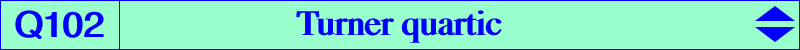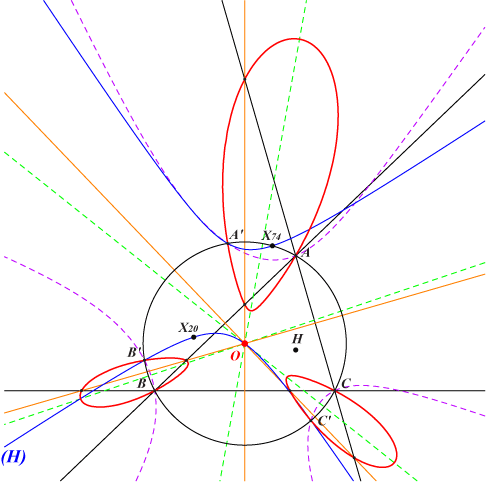too complicated to be written here. Click on the link to download a text file.X(3) other points belowThe locus of point M whose Turner line T(M) – see a reminder at the bottom of this page – passes through a given point P is generally a circular inversible nodal circum-quartic. It becomes bicircular if and only P = O and this gives the Turner quartic Q102. Thus, Q102 is a bicircular inversible nodal quartic with node O, the locus of point M whose Turner line T(M) passes through O. Q102 meets the circumcircle again at A', B', C' whose Simson lines pass through O. These points lie on the rectangular hyperbola (H) passing through X(3), X(20), X(74), X(2574), X(2575) which is the image of the Jerabek hyperbola under the translation that maps H onto O. Q102 contains 6 other points on the sidelines of ABC which are their intersections with the perpendicular bisectors of ABC other than the midpoints.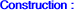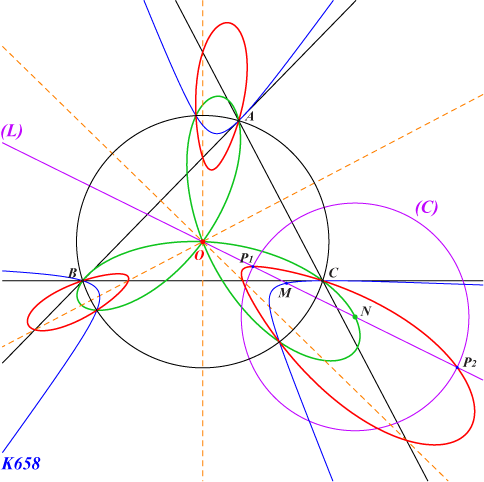First construct a point M on K658 which is the second intersection of the normal (L) at O to a variable circum-conic passing through O. The inverse N of M in the circumcircle lies on a trifolium already mentioned in the page K658. N is the center of a circle (C) orthogonal to the circumcircle. (C) and (L) meet at two points P1, P2 lying on Q102. If P1*, P2* are the isogonal conjugates of P1, P2 then the common Turner line of P1, P2 is the perpendicular bisector of P1*P2* and obviously contains O.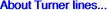The Turner line of a point P is a generalization of the Simson line but P does not have to be on the circumcircle. J. S. Turner defines it in 1924 as follows : if Q is the inverse of P and if L, M, N are the reflections of P in the sidelines of ABC then let X, Y, Z be the intersections of QL, QM, QN with BC, CA, AB respectively. These points X, Y, Z are collinear on the Turner line T(P) of P which is also the Turner line of Q. T(P) is also the perpendicular bisector of the isogonal conjugates P*, Q* of P, Q (Jean-Pierre Ehrmann). Hence T(P) contains the midpoint S of P*Q* which is actually the orthopole of the line PQ in ABC. S lies on the nine point circle.